# Parametric derivative

## Definition

### Algebraic definition

The parametric derivative$dy/dx$ for a parametric curve$x = f(t), y = g(t)$ at a point$t = t_0$ is given as follows, where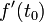$f'(t_0)$ and$g'(t_0)$ both exist and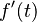$f'(t)$ is nonzero and of constant sign for an open interval around$t_0$: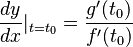$\! \frac{dy}{dx} |_{t = t_0} = \frac{g'(t_0)}{f'(t_0)}$

As a general function of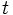$t$, the parametric derivative$dy/dx$ is defined as$g'(t)/f'(t)$.

NOTE: When calculating the general expression for the parametric derivative, before canceling any factors between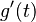$g'(t)$ and$f'(t)$, it is important to separate out the cases where that common value is zero. For any points where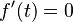$f'(t) = 0$, the parametric derivative is not defined (the ordinary derivative may still be defined, but we would need another method to calculate it).

MORE ON THE WAY THIS DEFINITION OR FACT IS PRESENTED: We first present the version that deals with a specific point (typically with a$\{ \}_0$ subscript) in the domain of the relevant functions, and then discuss the version that deals with a point that is free to move in the domain, by dropping the subscript. Why do we do this?
The purpose of the specific point version is to emphasize that the point is fixed for the duration of the definition, i.e., it does not move around while we are defining the construct or applying the fact. However, the definition or fact applies not just for a single point but for all points satisfying certain criteria, and thus we can get further interesting perspectives on it by varying the point we are considering. This is the purpose of the second, generic point version.

## Relation with ordinary derivative

### Parametric expressions aren't necessarily functions

The notation$dy/dx$ should be used only in the context that$y$ is a function of$x$, i.e., a single value of$x$ gives rise to a single value of$y$. Generally speaking, this is not guaranteed with parametric curves.

For instance, for a parametric curve$x = f(t), y = g(t)$,$y$ is expressible as a function of$x$ only if$g(t)$ is completely determined by$f(t)$. This is the case, for instance, when$f$ is a one-one function. But it's not always the case. Consider a circle:$x = \cos t, y = \sin t$

In this case, a single value of$x$, in most cases, corresponds to two values of$y$ that are negatives of each other. That's because if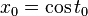$x_0 = \cos t_0$, we have also$x = \cos (-t_0)$, so both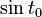$\sin t_0$ and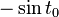$-\sin t_0$ work. The only case where$y$ is unique in terms of$x$ is when$x = 1$ and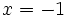$x = -1$. Geometrically, vertical secant lines intersect the upper semicircle and lower semicircle, and these are the two$y$-values for a given$x$-value.

### "Nice" parametric expressions define functions locally at most points

As described above, for a parametric curve,$y$ need not globally be a function of$x$. However, even in the presented example of a circle, for most$t_0$, if we restrict$t$ to a small enough open interval around$t_0$,$y$ is a function of$x$ for the part of the curve where$t$ is restricted to that interval. So it's "locally" a function. Differentiation being a local operation, it still makes sense to take the derivative at the point. The parametric derivative should therefore be understood as the derivative for the function obtained by taking the local restriction.

In the circle example, the only points at which$y$ is locally not a function of$x$ are the ones at the far left and far right:$x = 1$ and$x = -1$ (ironically, these are the only points with unique global$y$-values).

### If$f'(t)$ is nonzero (and of constant sign) for$t$ around$t_0$, the parametric expression defines a function locally around$t_0$

If$f'(t)$ is nonzero and of constant sign around$t_0$, then (depending on the sign)$f$ is an increasing or decreasing function around <mth>t_0[/itex]. In particular, within that open interval,$f$ is a one-one function, so no value of$x$ repeats. Thus locally$y$ is a function of$x$ on that interval.

NOTE: To deduce increasing/decreasing behavior, it is not enough to assume that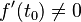$f'(t_0) \ne 0$. For a counterexample, see positive derivative at a point not implies increasing around the point.

NOTE 2: The assumption of constant sign is not necessary; it can be deduced from the derivative being nonzero. That's because derivative of differentiable function satisfies intermediate value property.

### The parametric derivative makes sense and is the correct expression when it is defined

We have now come full circle. In the case that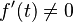$f'(t) \ne 0$ around$t_0$,$y$ is locally a function of$x$ around$t_0$. In such cases, if$g'(t_0)$ also exists, the expression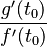$\frac{g'(t_0)}{f'(t_0)}$ gives the parametric derivative.

The proof that the parametric derivative expression is correct follows from the chain rule for differentiation. As established above,$y$ is locally a function of$x$ around$t_0$. Let's call this local function$h$. Locally around$t_0$, we have: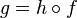$g = h \circ f$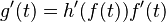$g'(t) = h'(f(t))f'(t)$

At$t = t_0$, we get: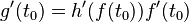$g'(t_0) = h'(f(t_0))f'(t_0)$

Rearranging, we get:$h'(f(t_0)) = \frac{g'(t_0)}{f'(t_0)}$

The left side is the expression we are trying to calculate, and the right side is the expression that we want to prove it to be.

### When the parametric derivative is undefined, the ordinary derivative may or may not be defined

Consider the example of the astroid as a parametric curve:$x = f(t) = \cos^3t, y = g(t) = \sin^3t$

The derivatives are as follows:$f'(t) = -3\cos^2t \sin t, g'(t) = 3\sin^2t \cos t$

The parametric derivative is therefore: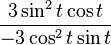$\frac{3\sin^2 t \cos t}{-3\cos^2 t \sin t}$

We can cancel the 3: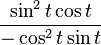$\frac{\sin^2 t \cos t}{-\cos^2 t \sin t}$

However, we should not cancel the$\sin t$ and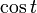$\cos t$ until we have isolated the cases where either of them is zero.

#### Cases of undefined parametric derivative

The cases where the derivative is undefined correspond precisely to the cases where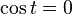$\cos t = 0$ or$\sin t = 0$, which correspond precisely to the integer multiples of$\pi / 2$. At these points, the parametric derivative is not defined. The actual point coordinates are (1,0), (0,1), (-1,0), and (0,-1).

#### Cases of defined parametric derivative

When the parametric derivative is defined, we can cancel the common factors from the numerator and denominator. After simplifying, we get: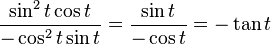$\frac{\sin^2 t \cos t}{-\cos^2 t \sin t} = \frac{\sin t}{-\cos t} = - \tan t$

#### Limiting behavior on undefined cases

When$t = 0$ or$t = \pi$, the limit of the parametric derivative expression (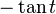$- \tan t$) equals zero. However, despite the limit existing, the parametric derivative is undefined at the point. In fact, the parametric curve is not locally a function at these points: geometrically, the$x$-coordinate doubles back, forming a horizontal cusp.

When$t = \pi /2$ or$t = 3\pi / 2$, the parametric derivative does not have a defined limit, with the left-hand and right-hand limits being opposite signs of infinity. Geometrically, we have vertical cusps at these points.

NOTE: In general, when the parametric derivative is not defined at a point, but there is a limit for it at that point, then the ordinary derivative, if defined, must equal that value.